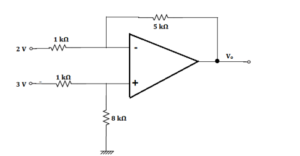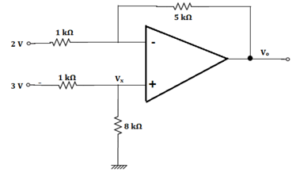Engineering Jobs   »   RVUNL ELECTRICAL QUIZ, RVUNL JE Admit...

# RVUNL’21 EE: Daily Practices Quiz 24-Aug-2021

RVUNL Recruitment 2021: The RVUNL exam date is released for a total of 1075 vacancies.
There are two parts for the RVUNL test, Part A and Part B.
Part A will have 60% weightage while Part B has 40% weightage out of the total marks in the online written exam.

RVUNL’21 EE: Daily Practices Quiz 24-Aug-2021
Each question carries 2 marks.
Negative marking: 0.5 mark
Total Questions: 06
Time: 08 min.

Q1. A 6-pole, 50 Hz, 1-phase induction motor runs at a speed of 900 r.p.m. The frequency of currents in the cage rotor will be
(a) 5 Hz, 50 Hz
(b) 5 Hz, 55 Hz
(c) 5 Hz, 95 Hz
(d) 55 Hz, 95 Hz
(e) None of the above

Q2. A parallel RLC circuit resonates at a frequency of 2 MHz. At a frequency 2.2 MHz, the nature of circuit impedance will be
(a) Resistive
(b) Inductive
(c) Capacitive
(d) Can’t say
(e) None of the above

Q3. If the Op-Amp in the figure is ideal, the output voltage Vo will be equal to(a) 1 V
(b) 6 V
(c) 14 V
(d) 17 V
(e) 13 V

Q4. A 40 KVA transformer has a core loss of 400 W and full-load copper loss of 800 W. The fraction of rated load at maximum efficiency is
(a) 50 %
(b) 62.3 %
(c) 70.7 %
(d) 100 %
(e) 86.6 %

Q5. The inductance of a high Q inductor can be measured using a
(a) Schering bridge
(b) Wein bridge
(c) Maxwell bridge
(d) Hay’s bridge
(e) All of the above

Q6. A series R-C circuit having R = 5 Ω and C = 100 μF has a time constant of
(a) 20 ×10^(-6) sec.
(b) 5 × 10^(-4) sec.
(c) 0.005 sec.
(d) 500 sec.
(e) 250 sec.

SOLUTIONS
S1. Ans.(c)
Sol. Synchronous speed for forward rotating flux, Ns = 120 f/P = 120 × 50/6 = 1000 r.p.m.
Therefore, slip for forward rotating flux is
s_f=s=(N_s-N)/N_s =(1000-900)/1000=0.1
∴ Rotor current frequency for sf is f’ = sf × f = 0.1 × 50 = 5 Hz.
Slip for backward rotating flux s_b =(2 – s) =(2 – 0.1) = 1.9. Therefore, rotor current frequency, f” = (2 – s) × f = 1.9 × 50 = 95 Hz.

S2. Ans.(c)
Sol. As given frequency is greater than resonant frequency for parallel RLC Circuit. So, nature will be capacitive and vice-versa for series RLC circuit.

S3. Ans.(b)
Sol. Given:
Op-amp is ideal.
feedback resistor (R_f) = 5 kΩ
R_1 = 1 kΩ
Inverting terminal voltage (V_1) = 2 V
non-Inverting terminal voltage (V_2) = 3 VAs input voltage is applied to both the inverting and non-inverting terminal, so the output voltage will be due to both.
We will calculate V_x using voltage divider rule,
V_x = 8/(1 + 8) × V_2 = 8/9 × 3
∴ V_x = 8/3 V
So, V_o= voltage due to inverting+ voltage due to non-inverting terminals
Now, V_o = -R_f/R_1 × V_1 + (1 + R_f/R_1 ) × V_x
⇒ V_o = -5/1 × 2 + (1 + 5/1) × 8/3
⇒ V_o = -10 + 6 × 8/3
⇒ V_o = -10 + 16
So, V_o = 6 V

S4. Ans.(c)
Sol. η=√(P_C/P_cufl )=√(400/800) ×100=70.7 %

S5. Ans.(d)
Sol. Bridges used for measurement of inductance:
Anderson bridge (Q<1).
Maxwell bridge (1<Q<10).
Hay bridge (Q>10).

S6. Ans.(b)
Sol. Time constant is
τ = RC = 5 × 100 × 10^(-6)= 5 × 10^(-4) sec.

Sharing is caring!

Thank You, Your details have been submitted we will get back to you.